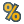You are viewing the RapidMiner Studio documentation for version 9.2 - Check here for latest version

#ANOVA (RapidMiner Studio Core)

## Synopsis

This operator is used for comparison of performance vectors. It performs an analysis of variance (ANOVA) test to determine the probability for the null hypothesis i.e. 'the actual means are the same'.

## Description

ANalysis Of VAriance (ANOVA) is a statistical model in which the observed variance in a particular variable is partitioned into components attributable to different sources of variation. In its simplest form, ANOVA provides a statistical test of whether or not the means of several groups are all equal, and therefore generalizes t-test to more than two groups. Doing multiple two-sample t-tests would result in an increased chance of committing a type I error. For this reason, ANOVA is useful in comparing two, three, or more means. 'False positive' or Type I error is defined as the probability that a decision to reject the null hypothesis will be made when it is in fact true and should not have been rejected. RapidMiner provides the T-Test operator for performing the t-test. Paired t-test is a test of the null hypothesis that the difference between two responses measured on the same statistical unit has a mean value of zero.

## Differentiation

###T-Test

Doing multiple two-sample t-tests would result in an increased chance of committing a type I error. For this reason, ANOVA is useful in comparing two, three, or more means.

## Input

•performance (Performance Vector)

This operator expects performance vectors as input it can have multiple inputs. When one input is connected, another performance input port becomes available which is ready to accept another input (if any). The order of inputs remains the same. The performance vector supplied at the first input port of this operator is available at the first performance output port of the operator.

## Output

•significance (ANOVA Significance)

The given performance vectors are compared and the result of the significance test is delivered through this port.

•performance (Performance Vector)

This operator can have multiple performance output ports. When one output is connected, another performance output port becomes available which is ready to deliver another output (if any). The order of outputs remains the same. The performance vector delivered at first performance input port of this operator is delivered at the first performance output port of the operator.

## Parameters

• alphaThis parameter specifies the probability threshold which determines if differences are considered as significant. If a test of significance gives a p-value lower than the significance level alpha, the null hypothesis is rejected. It is important to understand that the null hypothesis can never be proven. A set of data can only reject a null hypothesis or fail to reject it. For example, if comparison of two groups reveals no statistically significant difference between the two, it does not mean that there is no difference in reality. It only means that there is not enough evidence to reject the null hypothesis (in other words, the experiment fails to reject the null hypothesis). Range: real

## Tutorial Processes

### Comparison of performance vectors using statistical significance tests

Many RapidMiner operators can be used to estimate the performance of a learner or a preprocessing step etc. The result of these validation operators is a performance vector which collects the values of a set of performance criteria. For each criterion, the mean value and standard deviation are given. The question is how these performance vectors can be compared? Statistical significance tests like ANOVA or T-Test can be used to calculate the probability that the actual mean values are different. This Example Process performs exactly the same task.

This Example Process starts with a Subprocess operator which provides two performance vectors as output. Have a look at the inner operators of the Subprocess operator. The Generate Data operator is used for generating an ExampleSet. The Multiply operator is used for producing multiple copies of this ExampleSet. X-Validation operators are applied on both copies of the ExampleSet. The first X-Validation operator uses the Support Vector Machine (LibSVM) operator whereas the second X-Validation operator uses the Linear Regression operator in the training subprocess. The resultant performance vectors are the output of the Subprocess operator.

These performance vectors are compared using the T-Test and ANOVA operators respectively. The performance vectors and the results of the significance tests are connected to the result ports of the process and they can be viewed in the Results Workspace. Run the process and compare the results. The probabilities for a significant difference are equal since only two performance vectors were created. In this case the SVM is probably better suited for the data set at hand since the actual mean values are probably different. The SVM is considered better because its p-values is smaller than alpha which indicates a probably significant difference between the actual mean values.